JEE  >  VITEEE Maths Test - 2

# VITEEE Maths Test - 2

Test Description

## 40 Questions MCQ Test VITEEE: Subject Wise and Full Length MOCK Tests | VITEEE Maths Test - 2

VITEEE Maths Test - 2 for JEE 2023 is part of VITEEE: Subject Wise and Full Length MOCK Tests preparation. The VITEEE Maths Test - 2 questions and answers have been prepared according to the JEE exam syllabus.The VITEEE Maths Test - 2 MCQs are made for JEE 2023 Exam. Find important definitions, questions, notes, meanings, examples, exercises, MCQs and online tests for VITEEE Maths Test - 2 below.
Solutions of VITEEE Maths Test - 2 questions in English are available as part of our VITEEE: Subject Wise and Full Length MOCK Tests for JEE & VITEEE Maths Test - 2 solutions in Hindi for VITEEE: Subject Wise and Full Length MOCK Tests course. Download more important topics, notes, lectures and mock test series for JEE Exam by signing up for free. Attempt VITEEE Maths Test - 2 | 40 questions in 50 minutes | Mock test for JEE preparation | Free important questions MCQ to study VITEEE: Subject Wise and Full Length MOCK Tests for JEE Exam | Download free PDF with solutions
 1 Crore+ students have signed up on EduRev. Have you?
VITEEE Maths Test - 2 - Question 1

### The area of the region bounded by a2 y2 = x2 (a2 − x2) is

VITEEE Maths Test - 2 - Question 2

### The area bounded by the parabola y2=4ax and the straight line y=2ax is

Detailed Solution for VITEEE Maths Test - 2 - Question 2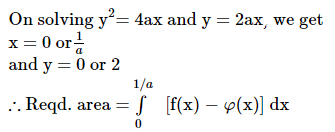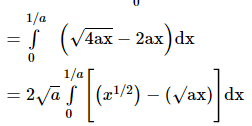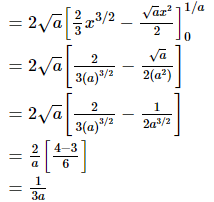VITEEE Maths Test - 2 - Question 3

### If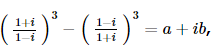then a and b are

VITEEE Maths Test - 2 - Question 4

Let z₁ and z₂ be nth roots of unity which subtend a right angle at the origin. Then n must be of the form

VITEEE Maths Test - 2 - Question 5

If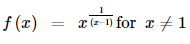and if f is continuous at x = 1 then f(1) =

VITEEE Maths Test - 2 - Question 6

The surface area of a cone including the base is 4π sq.ft., then the dimensions of the cone when the volume is maximum are

Detailed Solution for VITEEE Maths Test - 2 - Question 6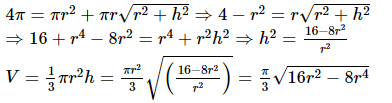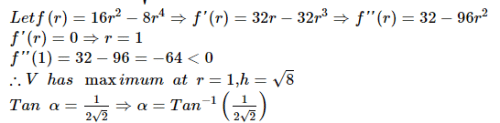VITEEE Maths Test - 2 - Question 7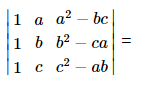VITEEE Maths Test - 2 - Question 8

If the function f is defined by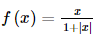hen at what points is f differentiable

VITEEE Maths Test - 2 - Question 9

Which one of the following differential equations represents the system of circles touching y-axis at the origin ?

VITEEE Maths Test - 2 - Question 10

The solution of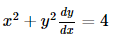is

VITEEE Maths Test - 2 - Question 11

The order of differential equation (d2y/dx2)3=(1+dy/dx)1/2 is

VITEEE Maths Test - 2 - Question 12

Solution of cos x dy/dx+y sin x =1 is

VITEEE Maths Test - 2 - Question 13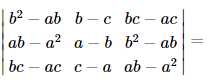Detailed Solution for VITEEE Maths Test - 2 - Question 13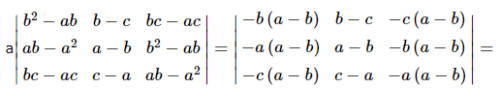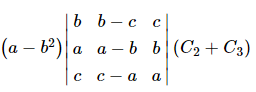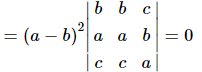VITEEE Maths Test - 2 - Question 14

If xy=e(x-y), (dy/dx)=

VITEEE Maths Test - 2 - Question 15

If f, g : R → R, f(x) = (x + 1)2 g(x) = x2 + 1 then (fog) (-3) =

VITEEE Maths Test - 2 - Question 16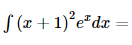VITEEE Maths Test - 2 - Question 17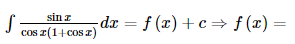VITEEE Maths Test - 2 - Question 18

tan⁻1(1/5)+tan⁻1(1/7)+tan⁻1(1/3)+tan⁻1(1/8)=

VITEEE Maths Test - 2 - Question 19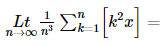VITEEE Maths Test - 2 - Question 20

The value of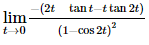is

VITEEE Maths Test - 2 - Question 21

Negation of the conditional , ' if it rains , I shall go to school ' is

VITEEE Maths Test - 2 - Question 22

If A is a square matrix and I is the unit matrix of the same order 3 x 3 , then A .(adj A)    is

VITEEE Maths Test - 2 - Question 23

Each coefficients in the equation ax2+bx+c=0 is determined by throwing an ordinary die. The probability that the equation will have equal roots is

VITEEE Maths Test - 2 - Question 24

A coin tossed until a head appears or until the coin has been tossed 5 times. If a head does not occur on the first two tosses, then the probability that the coin will be tossed 5 times is

VITEEE Maths Test - 2 - Question 25

Sum of all terms of a G.P. is 5 times the sum of odd terms. The common ratio is

VITEEE Maths Test - 2 - Question 26

If the side of a triangle are 13, 14, 15, then the radius of the incircle is

VITEEE Maths Test - 2 - Question 27

If A and B are such events that P(A)>0 and P(B)≠1, then P(A̅/B̅) is equal to

VITEEE Maths Test - 2 - Question 28

There are five roads from a village to a town. In how many ways can a villager return after reaching the town?

Detailed Solution for VITEEE Maths Test - 2 - Question 28

Permutation : -  A Permutation is an arrangement of all or part of a set of objects, with regard to the order of the arrangement.

For example, Suppose we have a set of three letters :A, B and C..... each possible arrangement would be an example of permutation.

Here in this question, there are 5roads leading to a town from a village.

He can go by 5 ways and can return by 4 ways

So the number of different ways in which a villager can go to the town and return back is 5×4=20

VITEEE Maths Test - 2 - Question 29

If B is a non-singular matrix and A is a square matrix, then det (B-1AB) =

VITEEE Maths Test - 2 - Question 30

If a,b are odd integers, the roots of the equation 2ax2+(2a+b)x+b=0, a≠0 are

VITEEE Maths Test - 2 - Question 31

If cov. (x, y) = 0, then ρ(x, y) equals

VITEEE Maths Test - 2 - Question 32

If cotθ=sin2θ(θ≠nπ), n ∈ Z then θ=

VITEEE Maths Test - 2 - Question 33

The number of real roots of equation x2-3|x|+2=0 is

VITEEE Maths Test - 2 - Question 34

A student obtain 75%, 80% and 85% in three subjects. If the marks of another subject are added, then his average cannot be less than

VITEEE Maths Test - 2 - Question 35

The equation of the plane which bisects the line joining (2,3,4) and (6,7,8) is

VITEEE Maths Test - 2 - Question 36

The equation of a circle with origin as a centre and passing through equilateral triangle whose median is of length 3a is

VITEEE Maths Test - 2 - Question 37

The equation of the sphere with centre (3, 6, -4) and touching the plane
2x - 2y - z - 10 = 0 is

Detailed Solution for VITEEE Maths Test - 2 - Question 37

As the required sphere touches the plane
2x - 2y - z - 10 = 0 .....(1)
∴ Radius of the required sphere = ⊥ from the center (3, 6, -4) of the required sphere to the plane (1)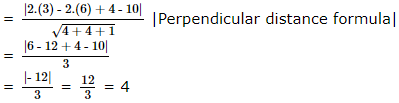∴ The required equation of the sphere is given by
(x - 3)2 + (y - 6)2 + (z + 4)2 = 16 (Central form)
⇒ x2 + 9 - 6x + y2 + 36 - 12y + z2 + 16 + 8z = 16
⇒ x2 + y2 + z2 - 6x - 12y + 8z + 45 = 0

VITEEE Maths Test - 2 - Question 38

The vertex of the parabola x2 + 8x + 12y - 8 = 0 is

VITEEE Maths Test - 2 - Question 39

Vectors 2i+3j-4k and ai+bj+ck are perpendicular, when

VITEEE Maths Test - 2 - Question 40

The moment of force F=i+2j+3k about the point 2i-j+k is

## VITEEE: Subject Wise and Full Length MOCK Tests

1 videos|3 docs|73 tests
 Use Code STAYHOME200 and get INR 200 additional OFF Use Coupon Code
Information about VITEEE Maths Test - 2 Page
In this test you can find the Exam questions for VITEEE Maths Test - 2 solved & explained in the simplest way possible. Besides giving Questions and answers for VITEEE Maths Test - 2, EduRev gives you an ample number of Online tests for practice

## VITEEE: Subject Wise and Full Length MOCK Tests

1 videos|3 docs|73 tests